# OECD Trust in government data analysis 4 - Simple linear regression using R - Trust in government ~ GDP, log(GDP).Photo by Dulana Kodithuwakku on Unsplash

This post is following of the above post.

Let's make log(capi) as l_capi.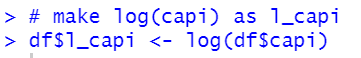Let's make a scatter plot for trust and l_capi.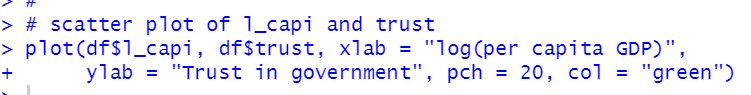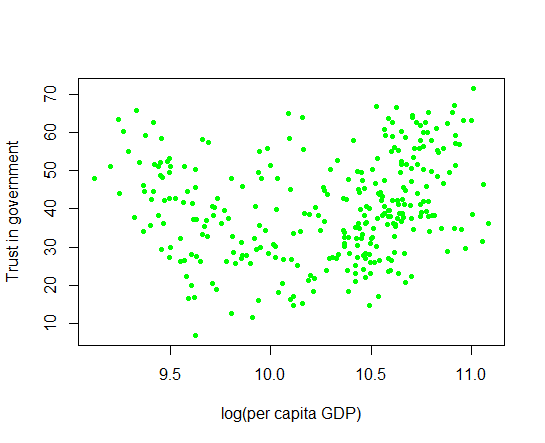It seems there is not clear relationship.

Then, I load broom package because I would like to use tidy() function with lm object.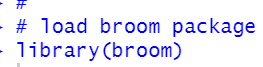All right, I will make some linear regression analysis, dependent variable is trust and independent variable is gdp, l_gdp, capi and l_capi. I will do it by year and by iso.
So, I will make 4 * 2 = 8 set of linear regression anaysis.

1. Independent variable = gdp by year.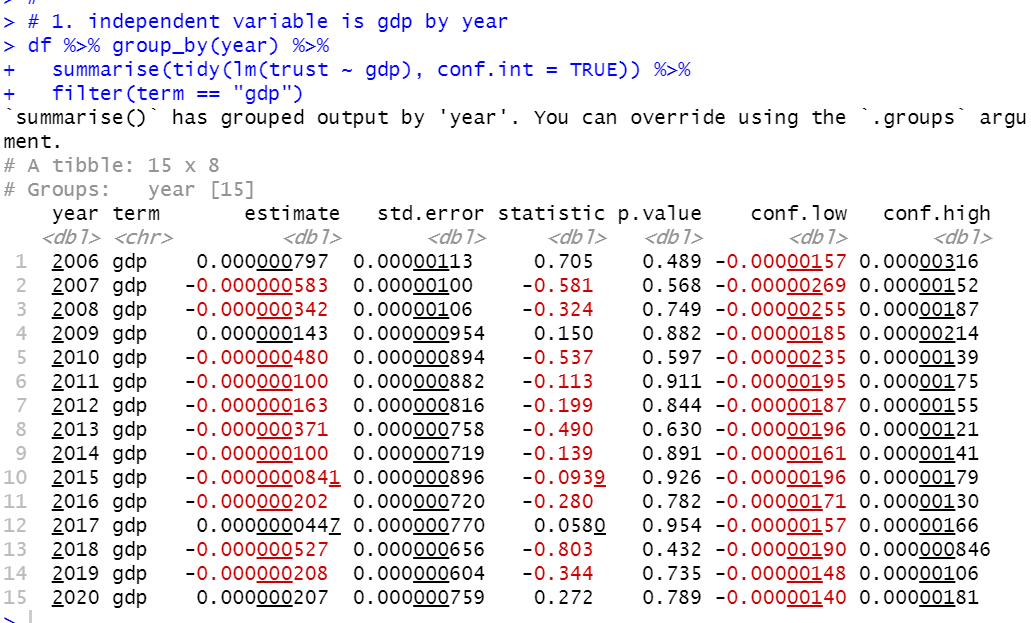For all year, from 2006 to 2020, p.value is greater than 0.05. So, by year regression, gpd is not significant variable fot trust.

2. Independent variable = gdp by iso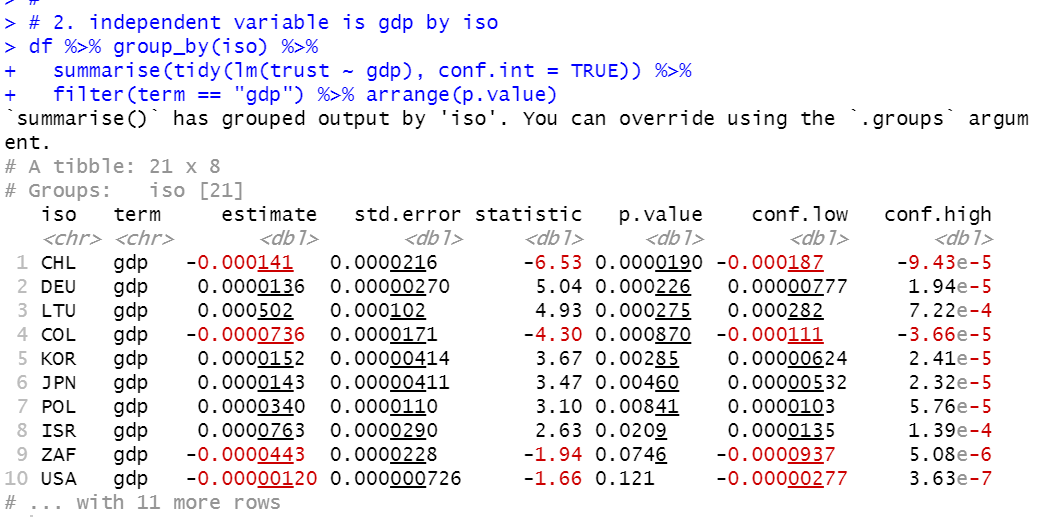Since there are 21 iso-s, I display the result p.value ascending order. CHL and COL hasve negative coefficient and others have positive coefficient. There are only 8 iso-s which have less than 0.05 p.value.

3. independent variable  = l_gdp by year.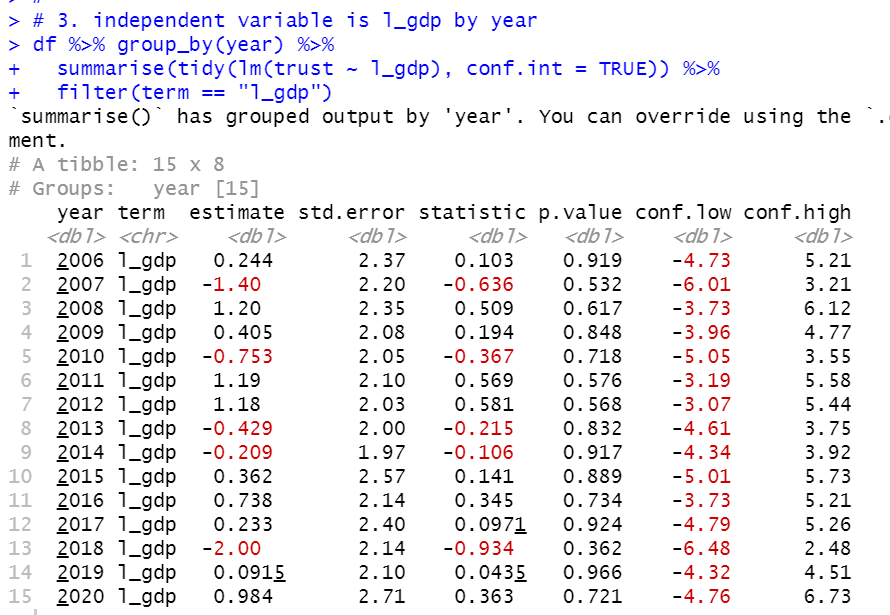We also see there is no year which have less than 0.05 p.value.

4. independent variable = l_gdp by iso.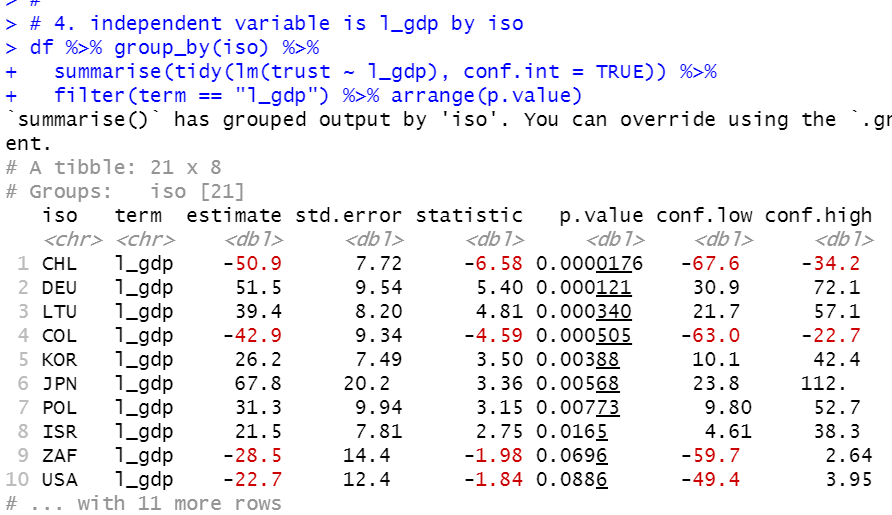Again, CHL and COL have negative coefficient with less than 0.05 p.value.

There are 8 iso-s which have less than 0.05 p.value.

I assume it is possible for GDP to affect Trust in government, but there must be other factors to affect it.

That's it in this post, next post I will see capi and l_capi.

Next post is

To see the 1st post